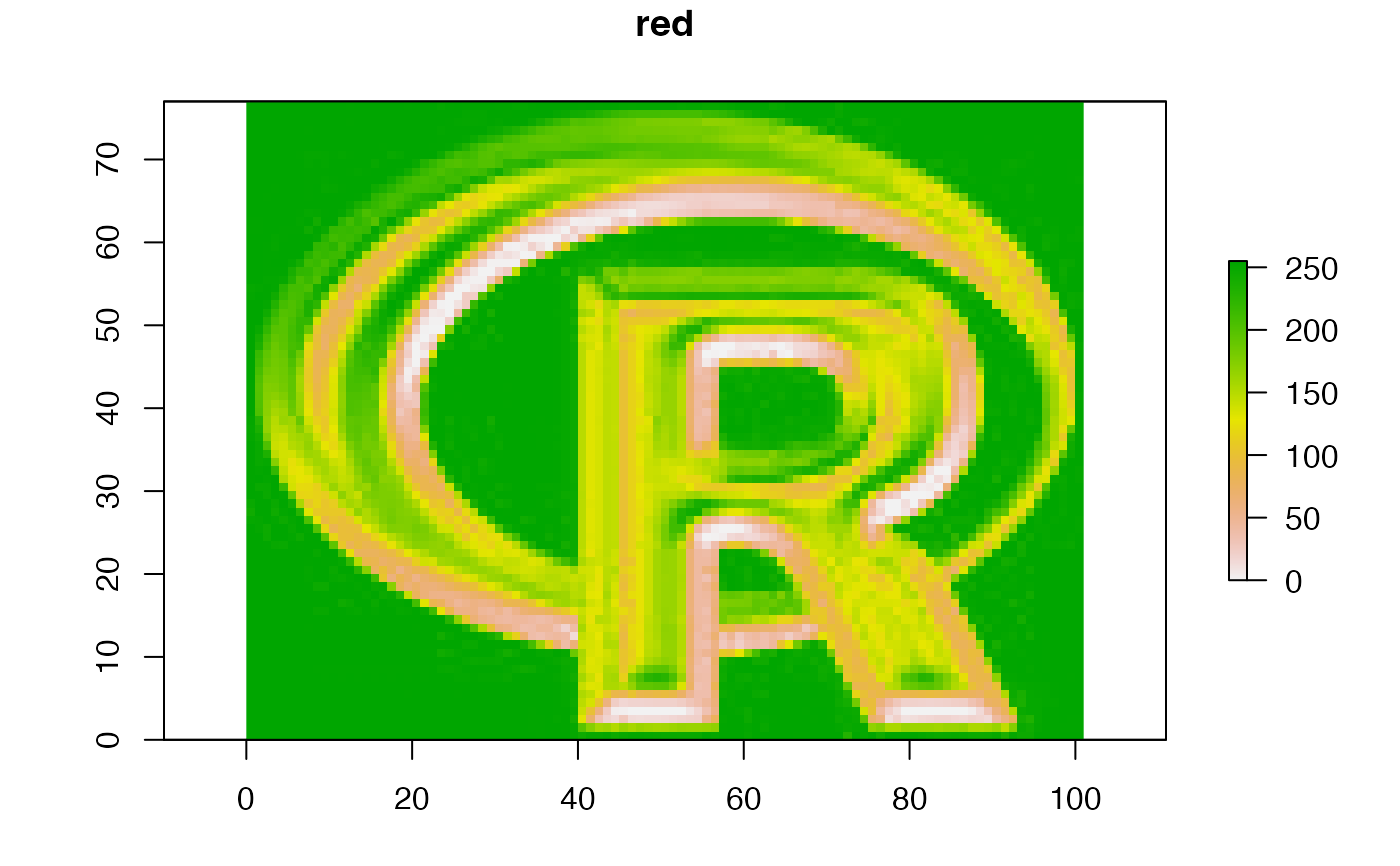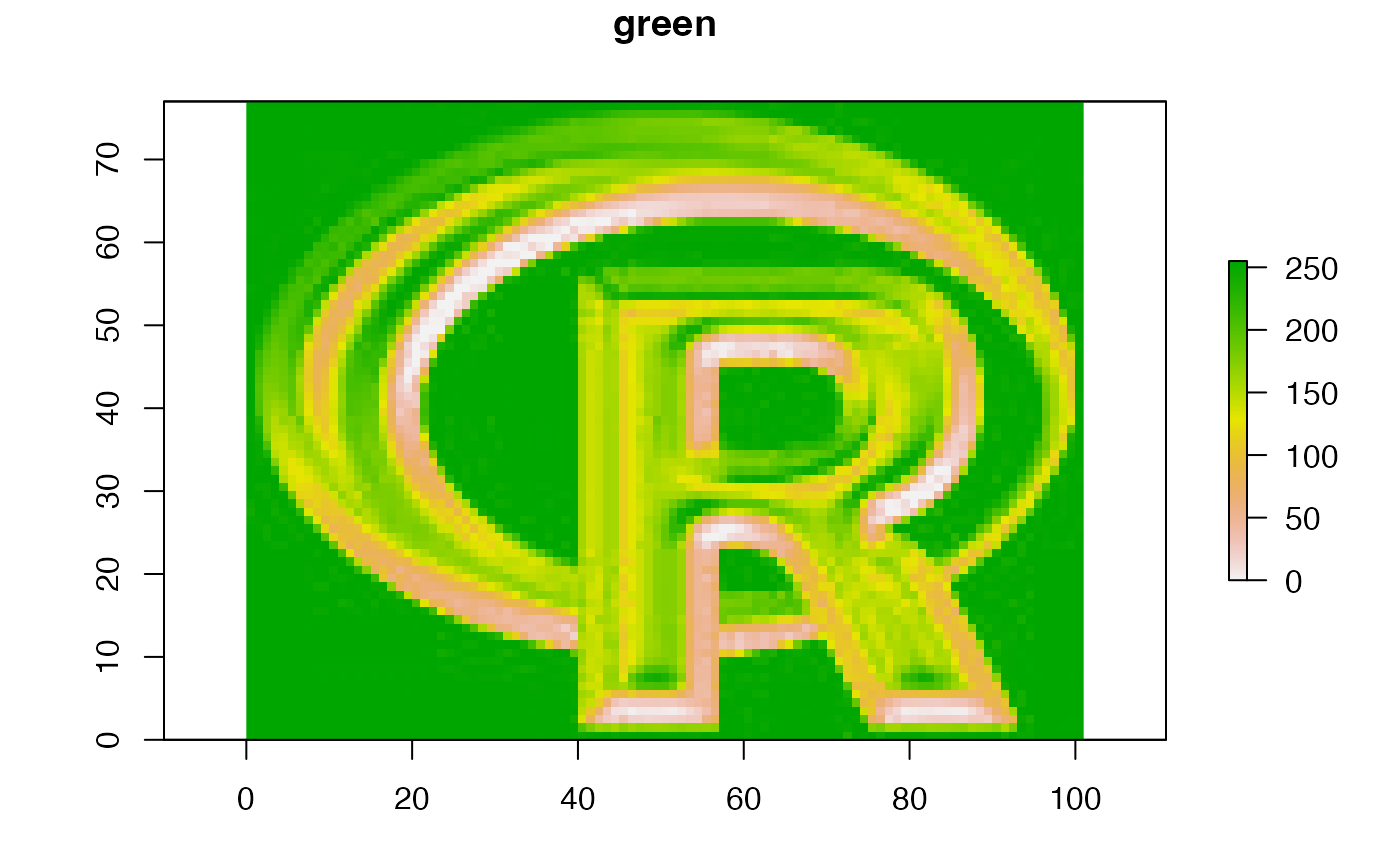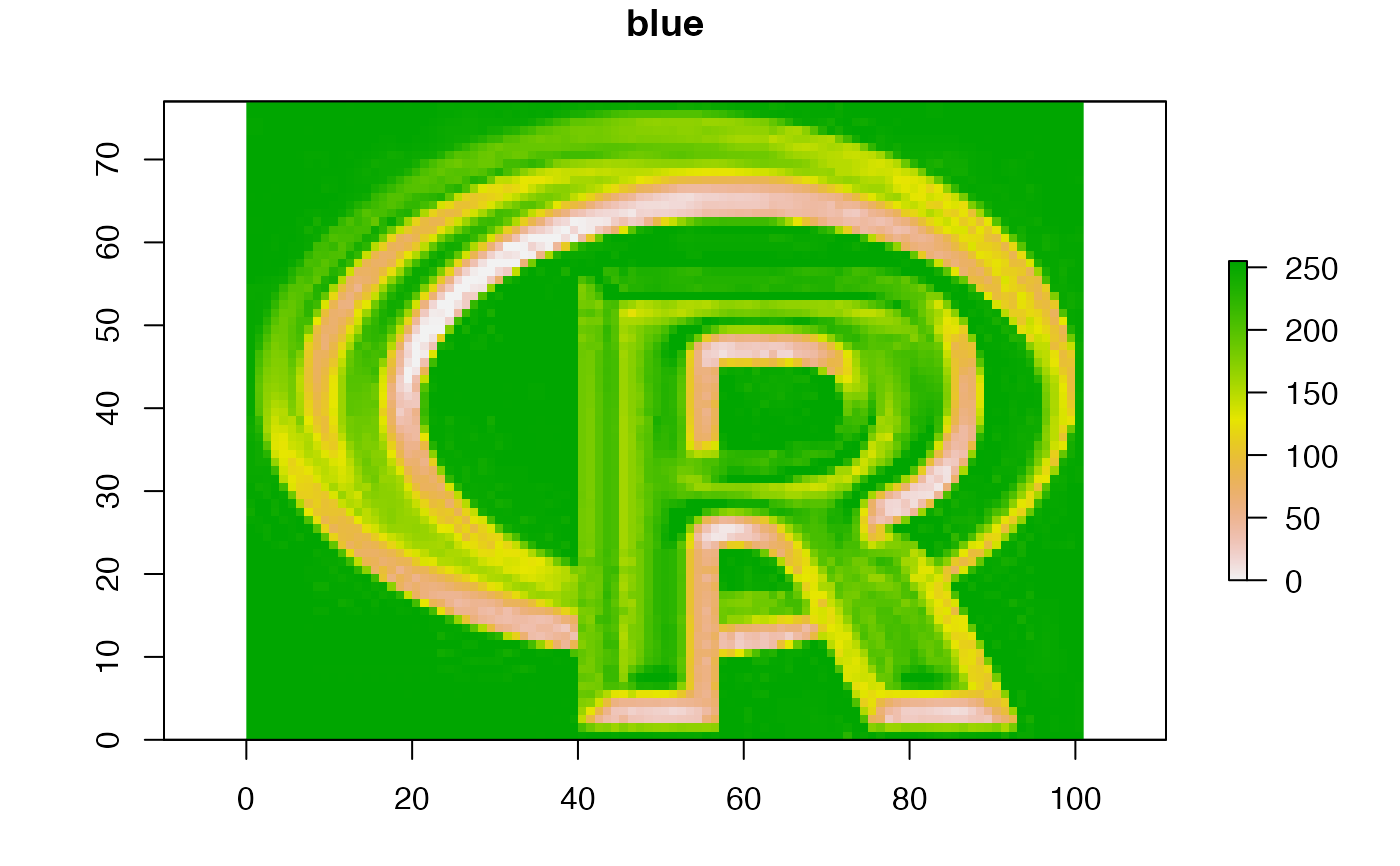Animate (sequentially plot) the layers of a RasterStack or RasterBrick* object to create a movie

# S4 method for RasterStackBrick
animate(x, pause=0.25, main, zlim, maxpixels=50000, n=10, ...)

## Arguments

x

Raster* object

pause

numeric. How long should be the pause be between layers?

main

title for each layer. If not supplied the z-value is used if available. Otherwise the names are used.

zlim

numeric vector of lenght 2. Range of values to plot

maxpixels

integer > 0. Maximum number of cells to use for the plot. If maxpixels < ncell(x), sampleRegular is used before plotting

n

integer > 0. Number of loops

...

Additional arguments passed to plot

## Value

None

plot, spplot, plotRGB
b <- brick(system.file("external/rlogo.grd", package="raster"))